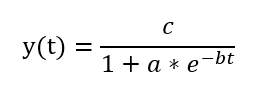# Logistic Growth Sigmoid Curve Chart Maker

The Logistic Growth Sigmoid Curve Chart Maker displays an S-shaped (sigmoid) growth curve for a user-specified set of logistic growth variables.

Logistic Growth Sigmoid Curve Chart Maker
Y Limiting Value (c):
Growth Rate (b):
Y Initial Value for t = 0
Number X Values Plotted on Chart:
Chart Title:

The logistic growth formula is:Where:

c is Y Limiting Value

a is (Y Limiting Value - Y Initial Value) / Y Initial Value

b is Growth Rate

## Related Chart Makers

Exponential Decay Calculator and Chart Maker

Exponential Growth Calculator and Chart Maker

Power Function Calculator and Chart Maker